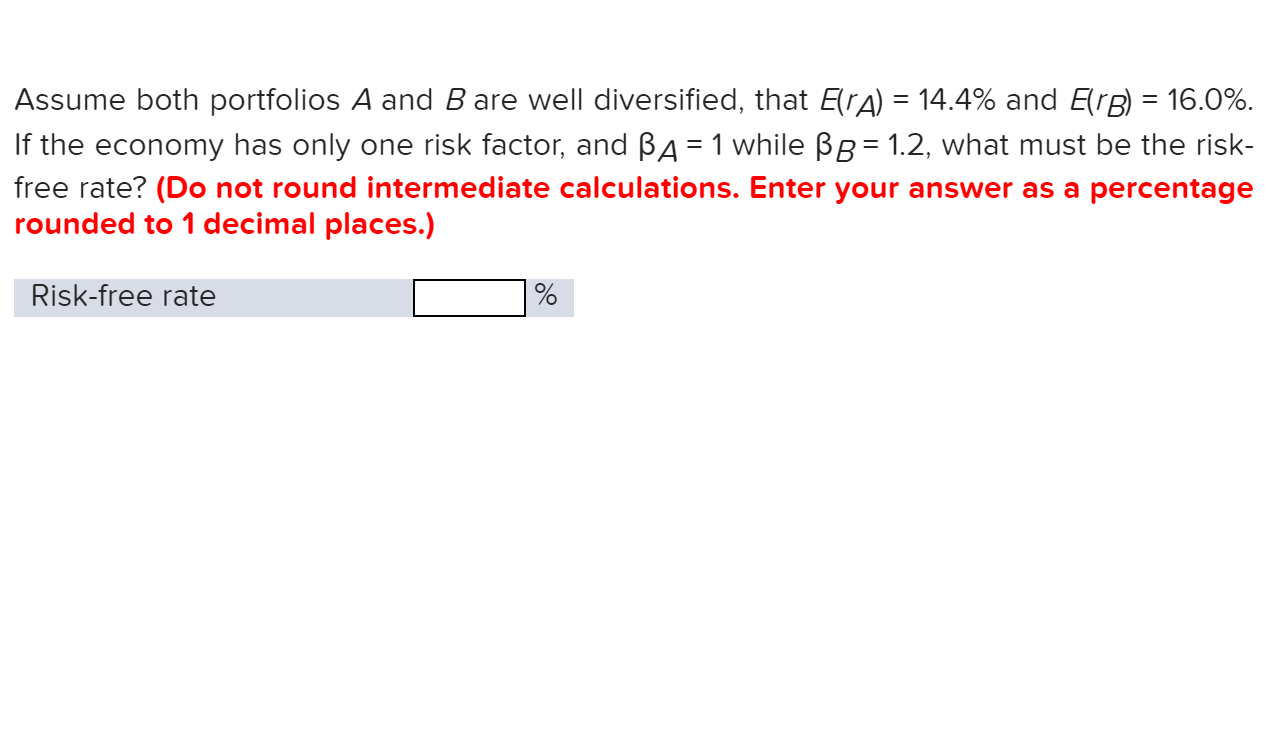# Assume both portfolios A and B are well diiversified, that ErA) = 14.4% and ErB 16.0%If the economy has only one risk factor, and BA 1 while BB= 1.2, what must be the risk-free rate? (Do not round intermediate calculations. Enter your answer as a percentagerounded to 1 decimal places.)Risk-free rate

Question
1 views

need helphelp_outlineImage TranscriptioncloseAssume both portfolios A and B are well diiversified, that ErA) = 14.4% and ErB 16.0% If the economy has only one risk factor, and BA 1 while BB= 1.2, what must be the risk- free rate? (Do not round intermediate calculations. Enter your answer as a percentage rounded to 1 decimal places.) Risk-free rate fullscreen
check_circle

Step 1

Under one factor model:

E(R) = Rf + Beta x Z where E(R) = expected return of a security, Rf = risk free rate, and Z = risk factor

Step 2

E(RA) = Rf + BetaA x Z

Hence, 14.4% = Rf + 1 x Z             - Eqn (1)

E(RB) = Rf + BetaB x ...

### Want to see the full answer?

See Solution

#### Want to see this answer and more?

Solutions are written by subject experts who are available 24/7. Questions are typically answered within 1 hour.*

See Solution
*Response times may vary by subject and question.
Tagged in